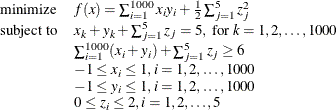### A Larger Optimization Problem

Consider the following larger optimization problem:The problem consists of a quadratic objective function, 1,000 linear equality constraints, and a linear inequality constraint. There are also 2,005 variables. The goal is to find a local minimum by using the ACTIVESET technique. This can be accomplished by issuing the following call to PROC OPTMODEL:

proc optmodel;
number n = 1000;
number b = 5;
var x{1..n} >= -1 <= 1 init  0.99;
var y{1..n} >= -1 <= 1 init -0.99;
var z{1..b} >=  0 <= 2 init  0.5;
minimize f = sum {i in 1..n} x[i] * y[i] + sum {j in 1..b} 0.5 * z[j]^2;
con cons1{k in 1..n}: x[k] + y[k] + sum {j in 1..b} z[j] = b;
con cons2: sum {i in 1..n} (x[i] + y[i]) + sum {j in 1..b} z[j] >= b + 1;
solve with NLP / algorithm=activeset logfreq=10;
quit;


The SAS output displays a detailed summary of the problem along with the status of the solver at termination, the total number of iterations required, and the value of the objective function at the local minimum. The summaries are shown in Figure 8.3.

Figure 8.3: Problem Summary and Solution Summary

The OPTMODEL Procedure

Problem Summary
Objective Sense Minimization
Objective Function f

Number of Variables 2005
Bounded Above 0
Bounded Below 0
Bounded Below and Above 2005
Free 0
Fixed 0

Number of Constraints 1001
Linear LE (<=) 0
Linear EQ (=) 1000
Linear GE (>=) 1
Linear Range 0

Performance Information
Execution Mode Single-Machine
Number of Threads 4

Solution Summary
Solver NLP
Algorithm Active Set
Objective Function f
Solution Status Optimal
Objective Value -996.5000004

Optimality Error 6.133411E-7
Infeasibility 5.4803721E-7

Iterations 5
Presolve Time 0.03
Solution Time 0.81

The SAS log shown in Figure 8.4 displays a brief summary of the problem that is being solved, followed by the iterations that are generated by the solver.

Figure 8.4: Progress of the Algorithm as Shown in the Log

 NOTE: Problem generation will use 4 threads. NOTE: The problem has 2005 variables (0 free, 0 fixed). NOTE: The problem has 1001 linear constraints (0 LE, 1000 EQ, 1 GE, 0 range). NOTE: The problem has 9005 linear constraint coefficients. NOTE: The problem has 0 nonlinear constraints (0 LE, 0 EQ, 0 GE, 0 range). NOTE: The OPTMODEL presolver removed 0 variables, 0 linear constraints, and 0 nonlinear constraints. NOTE: Using analytic derivatives for objective. NOTE: Using 2 threads for nonlinear evaluation. NOTE: The NLP solver is called. NOTE: The Active Set algorithm is used. Objective                          Optimality Iter             Value     Infeasibility             Error 0     -979.47500000        3.50000000        0.50000000 9     -996.49999988   0.0000002654239   0.0000000000116 NOTE: Optimal. NOTE: Objective = -996.4999999.